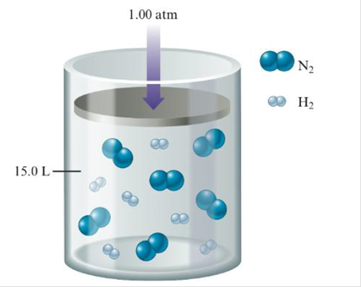Chapter 20, Problem 104CP

Chapter
Section
Textbook Problem

# Nitrogen gas reacts with hydrogen gas to form ammonia gas (NH3). Consider the following illustration representing the original reaction mixture in a 15.0 L container (the numbers of each molecule shown are relative numbers):Assume this reaction mixture goes to completion. The piston apparatus allows the container volume to change in order to keep the pressure constant at 1.00 atm. Assume ideal behavior and constant temperature.a. What is the partial pressure of ammonia in the container when the reaction is complete?b. What is the mole fraction of ammonia in the container when the reaction is complete?c. What is the volume of the container when the reaction is complete?

(a)

Interpretation Introduction

Interpretation: It is given that, nitrogen reacts with hydrogen gas (in a container) to produce ammonia gas. The volume of this mixture and total pressure is given. The partial pressure and mole fraction of ammonia in a container during completion of reaction is to be calculated. The volume of container during the completion of reaction is to be calculated.

Concept introduction: The volume is calculated using ideal gas law,

PV=nRT

The mole fraction of ammonia is calculated using the formula,

MolefractionofNH3=Moles of NH3Moles of N2+Moles of NH3

To determine: The partial pressure of ammonia in a container during completion of reaction.

Explanation

Given

Total pressure is 1.0atm .

The reaction of formation of ammonia is,

N2(g)+3H2(g)2NH3(g)

It is clear from the above equation that, one mole of nitrogen reacts with three moles of hydrogen to yield two moles of ammonia. In this reaction, hydrogen gas is limiting whereas nitrogen gas is in excess. Hence, the chemical equivalence is,

1molN23molH23molH22molNH3

In the given container, ammonia is formed due to combination of six moles of nitrogen gas and six moles of hydrogen gas. In this reaction, four moles of ammonia is produced due to consumption of two moles of nitrogen gas.

The number of moles of nitrogen gas consumed to form ammonia is calculated as,

MolesofN2required=Totalmolesofhydrogen×MolesofnitrogenMolesofhydrogen

Substitute the values of total moles of hydrogen, moles of nitrogen and hydrogen during ammonia formation in the above equation.

MolesofN2required=6molH2×1molN23molH2=2mol_

Remaining moles of nitrogen gas is calculated by subtracting total moles of nitrogen gas with moles of nitrogen consumed. Hence, remaining moles of nitrogen gas is,

6molN22molN2=4mol_

The number of moles of ammonia produced is calculated as,

MolesofNH3produced=Totalmolesofhydrogen×MolesofammoniaMolesofhydrogen

Substitute the values of total moles of hydrogen, moles of ammonia produced and hydrogen in the above equation.

MolesofNH3produced=Totalmolesofhydrogen×MolesofammoniaMolesofhydrogen=6molH2×2molNH33molH2=4mol_

The mole fraction of ammonia is 0

(b)

Interpretation Introduction

Interpretation: It is given that, nitrogen reacts with hydrogen gas (in a container) to produce ammonia gas. The volume of this mixture and total pressure is given. The partial pressure and mole fraction of ammonia in a container during completion of reaction is to be calculated. The volume of container during the completion of reaction is to be calculated.

Concept introduction: The volume is calculated using ideal gas law,

PV=nRT

The mole fraction of ammonia is calculated using the formula,

MolefractionofNH3=Moles of NH3Moles of N2+Moles of NH3

To determine: The mole fraction of ammonia in a container during completion of reaction.

(c)

Interpretation Introduction

Interpretation: It is given that, nitrogen reacts with hydrogen gas (in a container) to produce ammonia gas. The volume of this mixture and total pressure is given. The partial pressure and mole fraction of ammonia in a container during completion of reaction is to be calculated. The volume of container during the completion of reaction is to be calculated.

Concept introduction: The volume is calculated using ideal gas law,

PV=nRT

The mole fraction of ammonia is calculated using the formula,

MolefractionofNH3=Moles of NH3Moles of N2+Moles of NH3

To determine: The volume of container during the completion of reaction.

### Still sussing out bartleby?

Check out a sample textbook solution.

See a sample solution

#### The Solution to Your Study Problems

Bartleby provides explanations to thousands of textbook problems written by our experts, many with advanced degrees!

Get Started